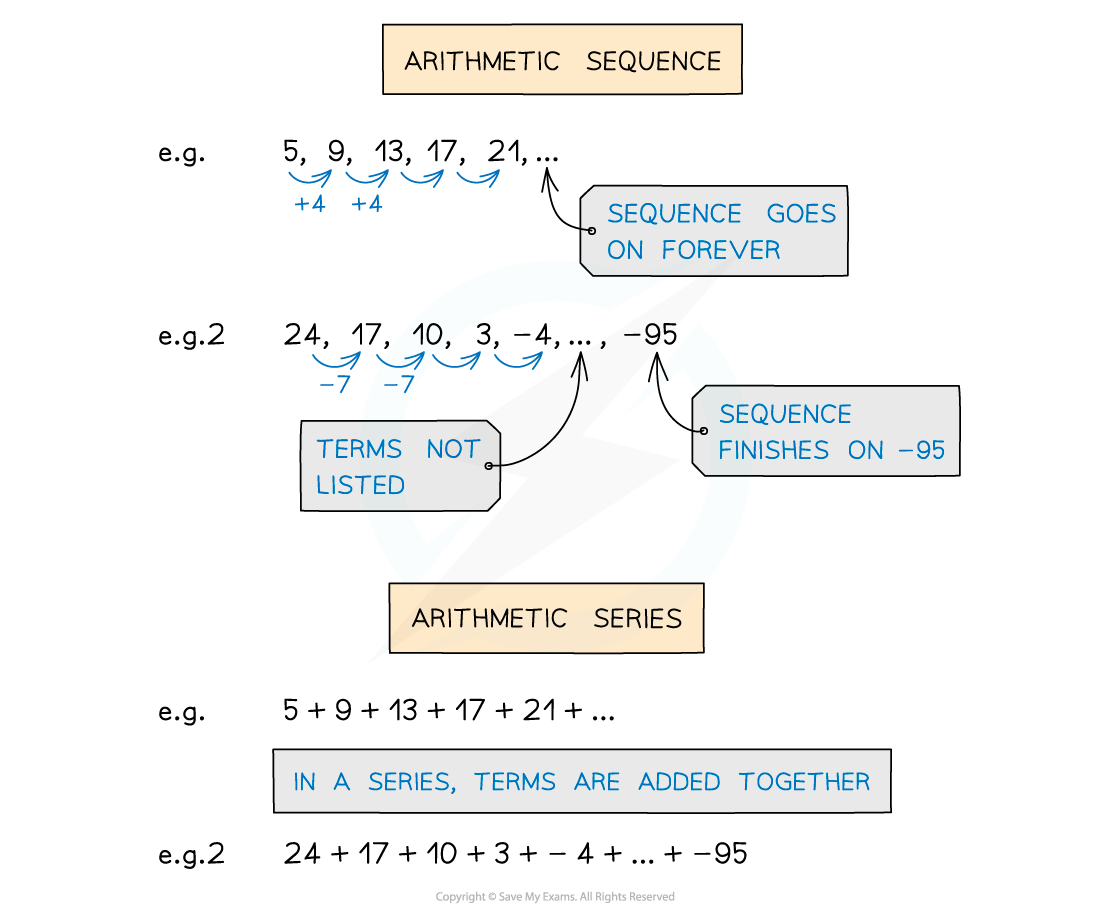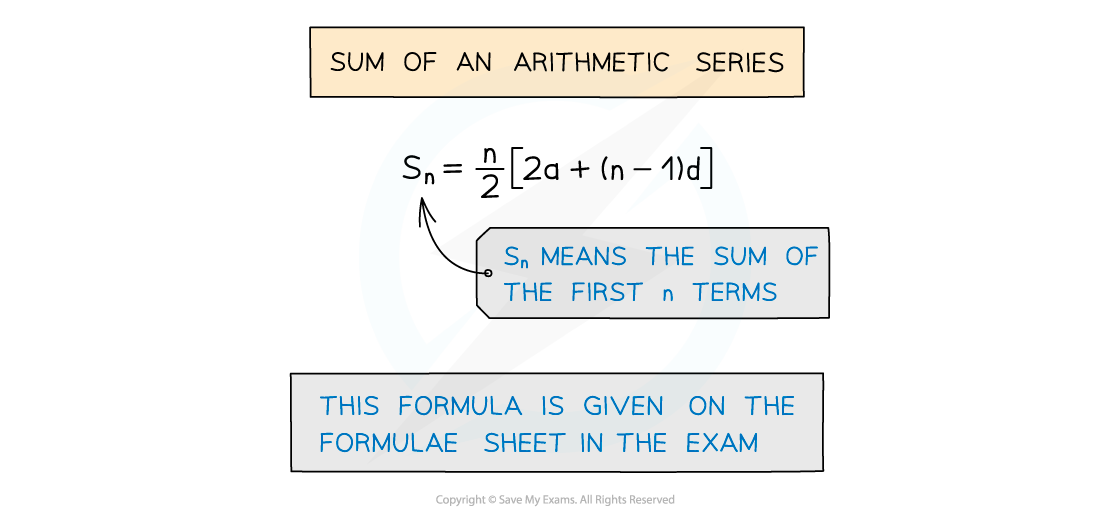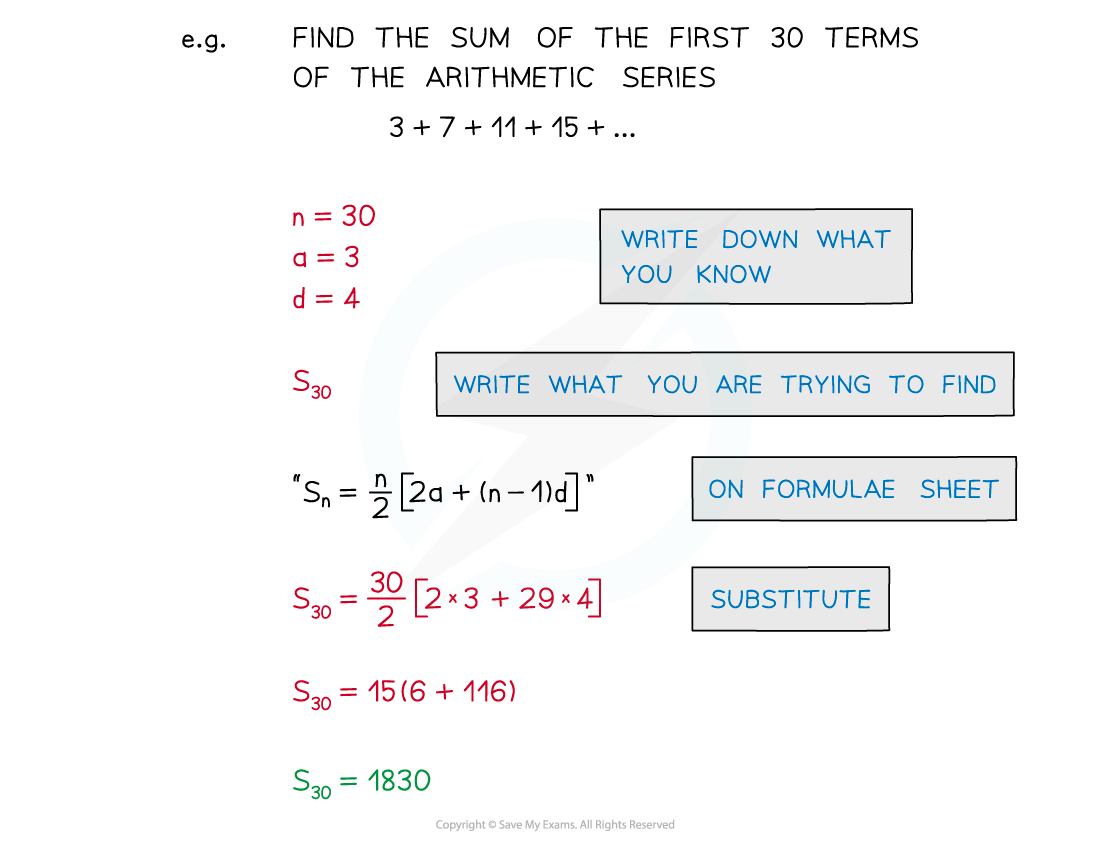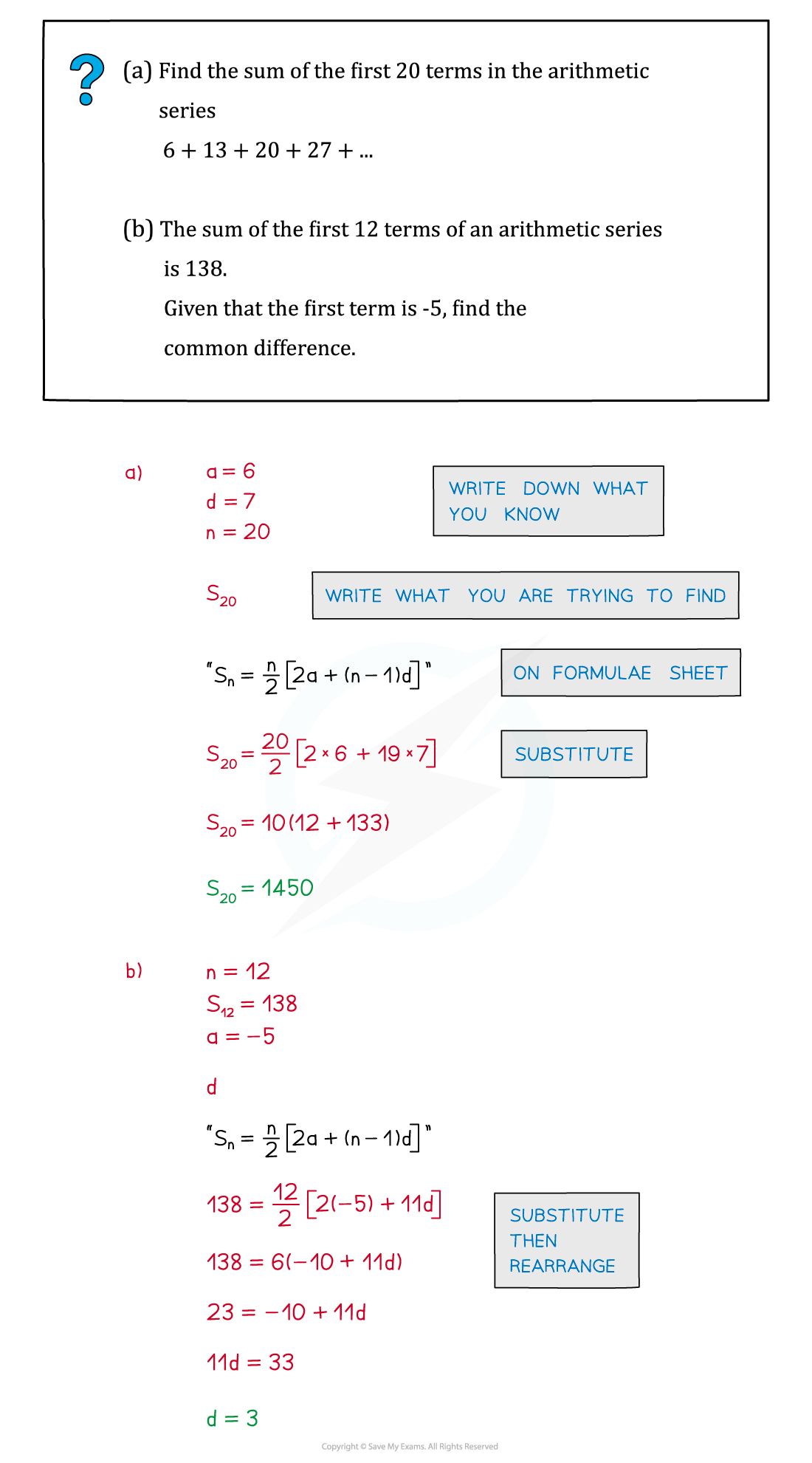# Edexcel IGCSE Maths 复习笔记 3.1.3 Arithmetic Sequences - Sum of n terms

Edexcel IGCSE Maths 复习笔记 3.1.3 Arithmetic Sequences - Sum of n terms

#### What is an arithmetic sequence or arithmetic series?

• Ensure you are familiar with Sequences – Basics and Linear• An arithmetic sequence is a sequence of numbers that increase or decrease by the same amount from one term to the next
• This amount is called the common difference
• eg. 5, 9, 13, 17, 21, ...  common difference of 4
• eg2. 24, 17, 10, 3, -4, ..., -95  common difference of -7

• An arithmetic series is where terms are added together
• eg. 5 + 9 + 13 + 17 + 21 + ...
• eg2. 24 + 17 + 10 + 3 + -4 + ... + -95• Lots of letters are used in sequences, make sure you are familiar with them
• a – the first term in an arithmetic series
• d – the common difference of an arithmetic series
• n – the number of terms in the arithmetic seriesSome series go on forever (ie.  have an infinite number of terms – but it could be that only the first 10 terms, say, are of interest, so n = 10)

• Sn is used for the sum of the first n terms of an arithmetic series

#### How do I find the sum of an arithmetic series?

• There is a formula for adding up the first n terms of an arithmetic series
• The formula is included on the formulae sheet• You do not need to where the formula comes from but, just for fun, here’s a hint
• To add up the numbers 1 to 10
• Write out the numbers1 2 3 4 5 6 7 8 9 10
• Write them backwards10 9 8 7 6 5 4 3 2 1
• Add up both lists11  11  11  11  11  11  11  11  11 11
• This is 10 × 11 = 110
• But this is twice the sum as two lots were added together
• So the sum of the numbers 1 to 10 is 110 ÷ 2 = 55#### Exam Tip

It is not necessarily Sn you’ll be asked to find in a question – any of a, d, n and S could be asked for.To avoid confusion always write down what you know and what you are trying to find.Remember that substituting known values into a formula first, then rearranging, is easier than the other way round!

#### Worked Example